• 1.线性情形 n = 50; x = linspace(40,70,n); y = .7*x + normrnd(0,5,size(x)); plot(x,y,'bx') hold on; [p,s] = polyfit(x,y,1);...[yfit,dy] = polyconf(p,x,s,'predopt','curve');...fill([x,fliplr(x)],[yfit-d
1.线性情形
n = 50;
x = linspace(40,70,n);
y = .7*x + normrnd(0,5,size(x));
plot(x,y,'bx')
hold on;
[p,s] = polyfit(x,y,1);
[yfit,dy] = polyconf(p,x,s,'predopt','curve');
fill([x,fliplr(x)],[yfit-dy,fliplr(yfit+dy)],[0.8706 0.9216 0.9804])
line(x,yfit,'color','r')
line(x,yfit-dy,'color','r','linestyle',':')
line(x,yfit+dy,'color','r','linestyle',':')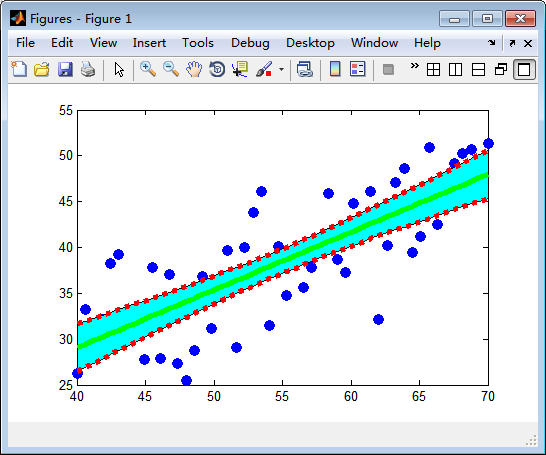2.非线性情形
x=0:0.1:1;
y=[-0.447 1.978 3.28 6.16 7.08 7.34 7.66 9.56 9.48 9.30 11.2];
A=polyfit(x,y,2)
z=polyval(A,x);
plot(x,y,'r*',x,z,'b')
hold on;
[p,s] = polyfit(x,y,2);
[yfit,dy] = polyconf(p,x,s,'predopt','curve');
fill([x,fliplr(x)],[yfit-dy,fliplr(yfit+dy)],[0.8706 0.9216 0.9804])
line(x,yfit,'color','r')
line(x,yfit-dy,'color','r','linestyle',':')
line(x,yfit+dy,'color','r','linestyle',':')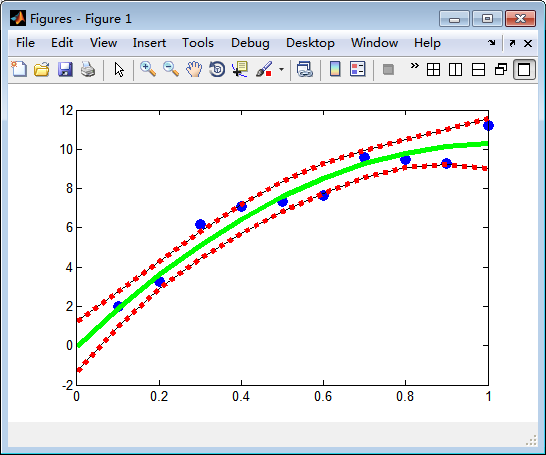展开全文• 三种方法 SPSS Matlab Sigmaplot 画置信区间线（或置信区间置信区间曲线 超实用
• 本代码主要利用MATLAB工具实现MATLAB——求解汽车平均行驶里程的置信区间，简单明了，易于理解
• MATLAB中与曲线拟合有关的函数主要有polyfit、polyval和polyconf。 1.polyfit：基于最小二乘法，用于曲线拟合的函数。 用法： （1）p=polyfit(x,y,n)。 其中，x和y为拟合数据向量，n为拟合多项式次数，缺省时...
    曲线拟合是已知离散点上的数据集，构造一个解析函数（其图形为一曲线），使在原离散点上尽可能接近给定的值。MATLAB中与曲线拟合有关的函数主要有polyfit、polyval和polyconf。

1.polyfit：基于最小二乘法，用于曲线拟合的函数。
    用法：
（1）p=polyfit(x,y,n)。
其中，x和y为拟合数据向量，n为拟合多项式次数，缺省时默认为1次。p中的系数按降幂排列，p的长度为 n+1。
（2）[p,s] = polyfit(x,y,n) 。
除p外还返回一个结构体s，可用作polyval的输入来获取误差估计值。

2.polyval：求n次多项式p在x处的值的函数。
    用法：
y1= polyval(p,x)。
输入变量p=[p0 p1 p2…pn]是一个长度为n+1的行向量，由一个多项式的系数组成，多项式系数按降幂排列，缺少的幂次要用0来补齐。常和polyfit搭配使用。

3.polyconf：对ployfit拟合曲线进行评价和置信区间估计的函数。
    用法：
[Y，DELTA]=polyconf（p，x，s，alpha）。
DELTA为polyfit所得的回归多项式在x处的预测值Y及预测值的显著性为1-alpha的置信区间。alpha缺省时默认是0.05。它假设polyfit函数数据输入的误差是独立正态的，并且方差为常数。p是polyfit函数的返回值；x和polyfit函数的x值相同；y和polyfit函数的y值相同。p和s可由polyfit函数的用法二获取。

x=0:51;
y=rand(1,51);
[p,s]=polyfit(x,y,4);
y1=polyval(p,x);
[yfit,dy]=polyconf(p,x,s,'predopt','curve');
h1=fill([x,fliplr(x)],[yfit-dy,fliplr(yfit+dy)],[0.8706 0.9216 0.9804]);
hold on
h2=plot(x,y1,'b','linewidth',2);
hold on
h3=plot(x,y,'r.','markersize',15);展开全文数据分析
• MATLAB 线性回归多项式拟合+预测区间、置信区间的绘制一、前言二、多项式拟合polyfit1、语法2、示例三、区间绘制四、整体源码五、思考六、参考博客 一、前言 现有一组数据：x、y x=[1 2 3 4 5 6 7 8 9 10]; y=[11 13...
MATLAB 线性回归多项式拟合+预测区间、置信区间的绘制一、前言二、多项式拟合polyfit1、语法2、示例三、区间绘制四、整体源码五、思考六、参考博客
一、前言
现有一组数据：x、y
x=[1 2 3 4 5 6 7 8 9 10];
y=[11 13 15 14 17 14 18 16 19 20];

对该数据进行线性回归（1次多项式拟合）并且绘制预测区间和置信度为95%的置信区间
拟合参数: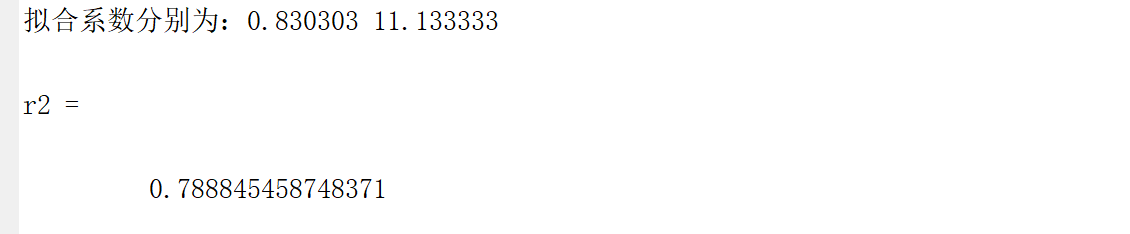拟合绘制：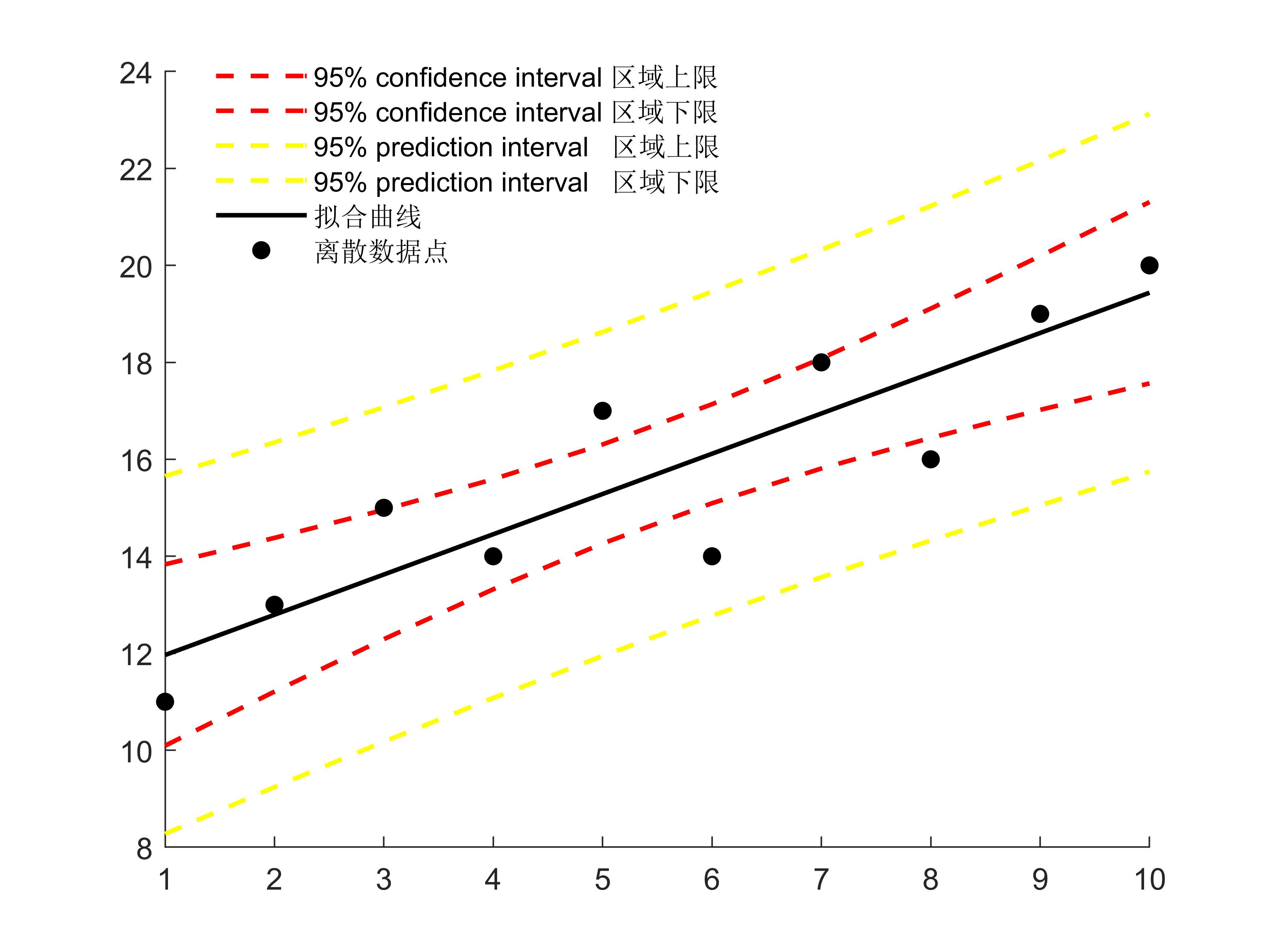二、多项式拟合polyfit
1、语法
 p=polyfit(x,y,n)：最小二乘法计算拟合多项式系数。x，y为拟合数据向量，要求维度相同，n为拟合多项式次数。返回p向量保存多项式系数，由最高次向最低次排列。

y=polyval(p,x)：计算多项式的函数值。返回在x处多项式的值，p为多项式系数，元素按多项式降幂排序。

具体语法可参考 ：【help-plyfit】
2、示例
x=[1 2 3 4 5 6 7 8 9 10];
y=[11 13 15 14 17 14 18 16 19 20];
a1=x;
a2=y;
[p,s]=polyfit(a1,a2,1);
fprintf("拟合系数分别为：%f %f\n",p(1),p(2));
y1= polyval(p,x);

三、区间绘制
这里提到：

置信区间估计(confidence interval estimate)：
利用估计的回归方程，对于自变量 x 的一个给定值 x0 ，求出因变量 y 的平均值的估计区间。

预测区间估计(prediction interval estimate)：
利用估计的回归方程，对于自变量 x 的一个给定值 x0 ，求出因变量 y 的一个个别值的估计区间。

代码如下：
% 95% prediction interval 计算：
[yfit,dy1] = polyconf(p,x,s);
% 95% confidence interval 计算：
[yfit,dy] = polyconf(p,a1,s,'predopt','curve');
hold on
%置信区域绘制
% fill([a1,fliplr(a1)],[yfit-dy,fliplr(yfit+dy)],[255/255 204/255 255/255],'EdgeColor','none');
% fill([a1,fliplr(a1)],[yfit-dy1,fliplr(yfit+dy1)],[255/255 204/255 255/255],'EdgeColor','none');
plot(a1,y1+dy,'r--',a1,y1-dy,'r--',a1,y1+dy1,'y--',a1,y1-dy1,'y--','LineWidth',1.5)

四、整体源码
%线性回归+置信区
%置信区间估计(confidence interval estimate)：利用估计的回归方程，对于自变量 x 的一个给定值 x0 ，求出因变量 y 的平均值的估计区间。
%预测区间估计(prediction interval estimate)：利用估计的回归方程，对于自变量 x 的一个给定值 x0 ，求出因变量 y 的一个个别值的估计区间。
clc;
clear;
%导入数据
x=[1 2 3 4 5 6 7 8 9 10];
y=[11 13 15 14 17 14 18 16 19 20];
% 数据排序，根据第一行的排列顺序进行整体排序
a=[x;y];
a1=a(1,:);
[a1,pos]=sort(a1);%左侧的a1是排列之后的第一行，pos是排序后的下标
a2=a(2,pos);      %a2,是排列好的第二行
% 多项式拟合
[p,s]=polyfit(a1,a2,1);
fprintf("拟合系数分别为：%f %f\n",p(1),p(2));
y1= polyval(p,a1);
% 95% prediction interval 计算：
[yfit,dy1] = polyconf(p,x,s);
% 95% confidence interval 计算：
[yfit,dy] = polyconf(p,a1,s,'predopt','curve');
hold on
%置信区域绘制
% fill([a1,fliplr(a1)],[yfit-dy,fliplr(yfit+dy)],[255/255 204/255 255/255],'EdgeColor','none');
% fill([a1,fliplr(a1)],[yfit-dy1,fliplr(yfit+dy1)],[255/255 204/255 255/255],'EdgeColor','none');
plot(a1,y1+dy,'r--',a1,y1-dy,'r--',a1,y1+dy1,'y--',a1,y1-dy1,'y--','LineWidth',1.5)
hold on
plot(a1,y1,'k','linewidth',1.5)
hold on;
scatter(x(1,1:10),y(1:10),'k','fill');
%R2
r2 = 1 - (sum((y1 - a2).^2) / sum((a2 - mean(a2)).^2))

五、思考

我在代码中增添了a1、a2，它们的作用是保证x、y数据的顺序性，对x进行排序，对应的y跟着x的排序进行变动。这样可以保证使用fill函数时能够得到我们想要的效果（填充满区域）。

六、参考博客
【1】、Matlab绘制带置信区间的拟合曲线
【2】、误差分析计算公式及其 matlab 代码实现(mse、mape、rmse等)
【3】、matlab曲线拟合 函数 用法以及例子（polyfit）
【4】、Matlab的拟合工具箱的误差参数说明
【5】、什么是预测区间和置信区间
欢迎各位留言交流！！！

同时，如果各位在作图方面有需求 可以闲鱼搜索用户：Man小洁，欢迎交流。


展开全文数据分析github
• MATLAB中与曲线拟合有关的函数主要有polyfit、polyval和polyconf。01polyfit：基于最小二乘法，用于曲线拟合的函数。用法：(1)p=polyfit(x,y,n)。其中，x和y为拟合数据向量，n为拟合多项式次数，缺省时默认为1次。p...


曲线拟合是已知离散点上的数据集，构造一个解析函数(其图形为一曲线)，使在原离散点上尽可能接近给定的值。MATLAB中与曲线拟合有关的函数主要有polyfit、polyval和polyconf。01polyfit：基于最小二乘法，用于曲线拟合的函数。用法：(1)p=polyfit(x,y,n)。其中，x和y为拟合数据向量，n为拟合多项式次数，缺省时默认为1次。p中的系数按降幂排列，p的长度为 n+1。(2)[p,s] = polyfit(x,y,n) 。除p外还返回一个结构体s，可用作polyval的输入来获取误差估计值。02polyval：求n次多项式p在x处的值的函数。用法：y1= polyval(p,x)。输入变量p=[p0 p1 p2…pn]是一个长度为n+1的行向量，由一个多项式的系数组成，多项式系数按降幂排列，缺少的幂次要用0来补齐。常和polyfit搭配使用。03polyconf：对ployfit拟合曲线进行评价和置信区间估计的函数。　用法：[Y，DELTA]=polyconf(p，x，s，alpha)。DELTA为polyfit所得的回归多项式在x处的预测值Y及预测值的显著性为1-alpha的置信区间。alpha缺省时默认是0.05。它假设polyfit函数数据输入的误差是独立正态的，并且方差为常数。p是polyfit函数的返回值；x和polyfit函数的x值相同；y和polyfit函数的y值相同。p和s可由polyfit函数的用法二获取。代码x=0:30;y=xlsread('C:\Users\Desktop\data.xlsx');[p,s]=polyfit(x,y,4);y1= polyval(p,x);[yfit,dy] = polyconf(p,x,s,'predopt','curve');h1=fill([x,fliplr(x)],[yfit-dy,fliplr(yfit+dy)],[0.8706 0.9216 0.9804]); hold onh2=plot(x,y1,'b','linewidth',2);  hold onh3=plot(x,y,'r.','markersize',15);  图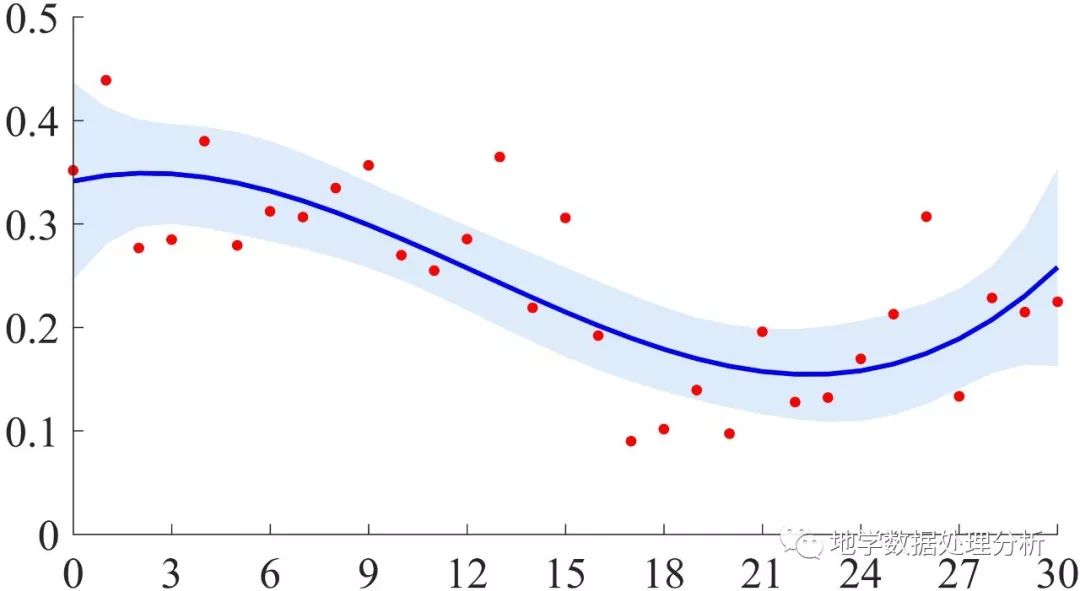▼更多精彩推荐，敬请关注我们▼展开全文• I want to plot some confidence interval graphs in MATLAB but I don't have any idea at all how to do it. I have the data in a .xls file.Can someone give me a hint, or does anyone know commands for plot...
• matlab的双y轴网上有很多方法，但是带置信区间的双y轴就很少了，并且由于网上给的例子一般都是使用红蓝两色，对于只想使用黑色或者灰色的俺们来说太鲜艳啦~    上图为使用matlab绘制的双y轴带置信区间的曲线。...string c
• 本文档参考：https://www.mathworks.com/help/stats/polyconf.html 代码如下： xdata = feat(:,1);% x值 替换自己的 ydata = bpt(:,1); % y值 替换自己的 degree = 2; % Degree of the fit ...[p,S] ...
• function interval=ci(x,confidence,dim);% interval=ci(x,confidence,dim);%% 90%, 95% or 99%confidence interval of a vectoror 2d matrix% Optional dim refers to dimension and must be 1 or 2 (default is1)....
• 实验九统计推断一、汽油㈠问题描述据说某地汽油的价格是115...(3)如何给出1月和2月汽油价格差的置信区间㈡简要分析本题要求在总体方差未知的情况下对总体均值进行假设检验，并求置信区间。1、假设检验H0：u=u0，H1：...
• 最近需要画带有置信区间的拟合图，其中在matlab的doc中搜索“Confidence and Prediction Bounds”，出现了两种置信区间。 原博客：https://www.cnblogs.com/100thMountain/p/5539024.html 置信区间估计 (confidence ...
• 使用fill函数绘制置信范围，用plot函数绘制数据点，来创建具有置信范围的折线图。使用点语法object.PropertyName来自定义绘图的外观。x 注：本文根据MATLAB官网内容修改而成。我推荐给你以下的视频教程，特点是没有...
• 前言 最近，自己接的小项目涉及到了离散数据点的曲线拟合和拟合后的置信区间绘制。自己在借鉴书籍和优秀博主的技术贴的同时，进行了一番学习和...【1】、Matlab绘制带置信区间的拟合曲线 【2】、误差分析计算公式及其数据分析
• MATLAB中与曲线拟合有关的函数主要有polyfit、polyval和polyconf。01polyfit：基于最小二乘法，用于曲线拟合的函数。用法：(1)p=polyfit(x,y,n)。其中，x和y为拟合数据向量，n为拟合多项式次数，缺省时默认为1次。p...
• 均值的置信区间对可以计算置信度为1-α的置信区间，称为均值的置信区间。 在predict()中加选项interval="confidence",用level=指定置信度，可以计算均值的置信区间。 如predict( lmrst02, interval="confidence", ...
• 使用fill函数绘制置信范围，用plot函数绘制数据点，来创建具有置信范围的折线图。使用点语法object.PropertyName来自定义绘图的外观。...%构造正反向的x值，作为置信区间的横坐标值 yconf = [y...
• 统计中经常会遇到求置信区间、置信区域（如置信椭圆、置信椭球）等，有时候需要把置信区域画出来，这样看起看更为直观，下面结合具体案例介绍调用自编函数ConfidenceRegion绘制置信区域。 【例1】绘制置信区间。...
• %使用 fill 函数绘制置信范围，同时使用 plot 函数绘制数据点，以此方式创建含有置信范围的绘图 x= 0:0.2:10; y = besselj(0, x);%贝塞尔函数 xconf = [x x(end:-1:1)] ;%一个来回;2列数 yconf = [y+0.15 y(end:-...
• ***************************************统计中经常会遇到求置信区间、置信区域(如置信椭圆、置信椭球)等，有时候需要把置信区域画出来，这样看起看更为直观，下面结合具体案例介绍调用自编函数ConfidenceRegion...
• 我用fminsearch 进行了常微分方程组参数优化 现想知道参数置信区间 所以改用lsqnonlin进行单参数拟合 初值是我已经得到的优化结果 但是拟合显示local minimum 而且ci = nlparci(k_opt,residual,'Jacobian',jacobian)...
• 本文介绍了MATLAB软件的normfit()函数在求解正态总体参数的区间估计中的长处和短处，结合实例编写了MATLAB程序求解标准差σ已知时均值μ的置信区间和均值μ已知时标准差σ的置信区间，弥补了normfit()函数在该方面的...
• We have following linear regression: y ~ b0 + b1 * x1 + b2 * ... I know that regress function in Matlab does calculate it, but numpy's linalg.lstsq doesn't (https://docs.scipy.org/doc/numpy-dev/user/n...
• % 方差类型为等方差 % 调用ttest2函数作两个正态总体均值的比较检验， % 返回变量h，检验的p值，均值差的置信区间muci，结构体变量stats >> [h,p,muci,stats] = ttest2(x,y,alpha,tail,vartype) 例6、首先用产生......

# matlab置信区间matlab 订阅